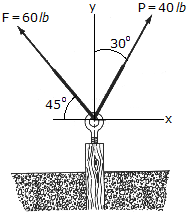# Engineering Mechanics - Force Vectors - Discussion

### Discussion :: Force Vectors - General Questions (Q.No.4)

4.Determine the magnitude and direction of the resultant force.

 [A]. R = 80.3 lb,= 106.2° CCW [B]. R = 80.3 lb,= 73.8° CCW [C]. R = 72.1 lb,= 63.6° CCW [D]. R = 72.1 lb,= 116.4° CCW

Explanation:

No answer description available for this question.

 Sajun said: (Jan 10, 2012) Its simple. By adding the why components and x components vectoricaly we are able to get the answer.

 Dan said: (Jan 22, 2012) Are you sure A is the right answer? you might want to find the tangent again.

 Sathish Uthayasuriyan said: (Apr 10, 2012) If we take tangent of these two the answer will be Option B.

 Snehasish said: (Jun 19, 2012) CCW means angle makes from west corner (-ve X-axis) i.e. 180 - 73.77 = 106.228 ~ 106.2.

 Cj Manglalan said: (Jul 22, 2012) CCW I THINK IT MEANS IT ROTATES Counter ClockkWise FOR F: F1x= (60 lb) (cos 45)= 42.426 f1y= 42.426 (sin 45=cos45) or (60 lb)(sin 45) FOR P: p1x= (40lb)(sin 30) = 20 p1y= (40lb)(cos 30) =34.641 sumamtion of forces along x =42.426 + 20 = -22.426 summation of forces along y =42.426 + 34.641 = 77.067 for R: R= square root of (((-22.426)square)+ ((77.067)square)) for angle: =arc tangent (y/x) =arc tangent (77.067/-22.426) = -73.8 since the angle is negative you will subtract it to 180 the angle is 180-73.8 = 106.2

 D.Mani Obul Reddy said: (Apr 15, 2014) Horizontal components = 60cos45 - 40sin30 = 22.426. Vertical components = 60sin45+40cos30 = 77.067. R = SQUARE ROOT(22.426)^2+(77.067)^2 = 80.26. ANGLE = TAN inverse of(77.067/22.426) = 73.77. 180 - 73.77 = 106.2 ccw.

 Ng Kl said: (Sep 29, 2014) I calculated the answer is -7.78. (60 lb) (cos 45) is negative.

 Bob said: (Sep 26, 2015) Why do you have to minus 73.7 from 180?

 Krishna said: (Sep 2, 2016) @Mani Obul. Yes, why you subtracted 73.7 from 180?

 Sarvjeet Singh said: (Nov 5, 2016) R = 80.25 s/ direction = 73.72.

 Thato said: (Mar 17, 2017) @All. Try parallelogram method to get the answer.

 Toms said: (Aug 10, 2017) How would you solve the x and y components if you don't know the angles?

 Bright said: (Oct 14, 2017) Why is 60cos45 negative?